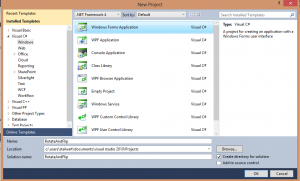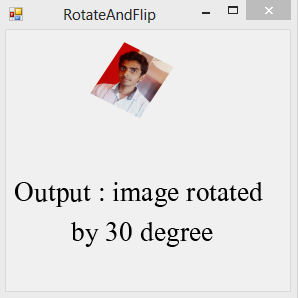# Rotate and Flip Images in Windows Form

By | September 12, 2012

One of my friends asked me how to rotate an image to a particular angle, so I just thought of writing an article on it. Below is the step-wise process on how to use the inbuilt functions for rotating and flipping the image.

Step 1: Create a blank windows form applicationStep 2: Add a pictureBox from the toolbox and add a source image to it.

Now comes the real part, using Image.RotateFlip we can rotate the image by standard angles(90,180,270 degrees) or flip the image horizontally or vertically.

The parameter of RotateFlip is System.Drawing.RotateFlipType, which specifies the type of rotation and flip to apply to the image.

e.g : pictureBox1.Image.RotateFlip(RotateFlipType.Rotate180FlipNone);

the above code will rotate the image in pictureBox1 by an angle of 180 degree.

Step 3: Now back to our code, add namespaces we will use here

using System.Drawing.Imaging;
using System.Drawing.Drawing2D;

Step 4: We will make a function RotateImg with two arguments one is the Bitmap Image and the other is the float angle. Code snippet is as follows :

public static Bitmap RotateImg(Bitmap bmp, float angle)
{
int w = bmp.Width;
int h = bmp.Height;
Bitmap tempImg = new Bitmap(w, h);
Graphics g = Graphics.FromImage(tempImg);
g.DrawImageUnscaled(bmp, 1, 1);
g.Dispose();
GraphicsPath path = new GraphicsPath();
Matrix mtrx = new Matrix();
mtrx.Rotate(angle);
RectangleF rct = path.GetBounds(mtrx);
Bitmap newImg = new Bitmap(Convert.ToInt32(rct.Width), Convert.ToInt32(rct.Height));
g = Graphics.FromImage(newImg);
g.TranslateTransform(-rct.X, -rct.Y);
g.RotateTransform(angle);
g.InterpolationMode = InterpolationMode.HighQualityBilinear;
g.DrawImageUnscaled(tempImg, 0, 0);
g.Dispose();
tempImg.Dispose();
return newImg;
}

Step 5: Now we have a function which will rotate and image to the arbitrary angle, I’m adding my code now to the form_load event so that as soon as the form loads my code gets executed.

Bitmap bitmap = (Bitmap)pictureBox1.Image;
pictureBox1.Image = (Image)(RotateImg(bitmap, 30.0f));

the above two line code will first convert the image in pictureBox1 to a Bitmap image and then it will call our function RotateImg to rotate the image to 30 degree (example).

Here is the snapshot of the output window.This site uses Akismet to reduce spam. Learn how your comment data is processed.